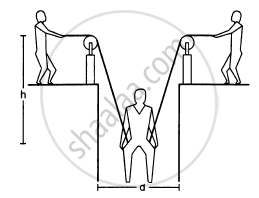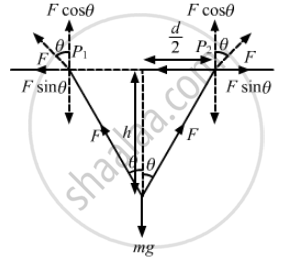# A Man Has Fallen into a Ditch of Width D and Two of His Friends Are Slowly Pulling Him Out Using a Light Rope and Two Fixed Pulleys as Shown in - Physics

Sum

A man has fallen into a ditch of width d and two of his friends are slowly pulling him out using a light rope and two fixed pulleys as shown in the following figure. Show that the force (assumed equal for both the friends) exerted by each friend on the road increases as the man moves up. Find the force when the man is at a depth h.#### Solution

(a) At any depth, let the ropes makes an angle θ with the vertical.From the free-body diagram,
Fcosθ + Fcosθ − mg = 0
2Fcosθ = mg

$\Rightarrow F = \frac{mg}{2cos\theta}$
As the man moves up, θ increases, i.e. cosθ decreases. Thus, F increases.
$\cos\theta = \frac{h}{\sqrt{\left( d/2 \right)^2 + h^2}}$
(b) When the man is at depth h,
$F = \frac{mg}{2\cos\theta}$
$= \frac{mg}{2\left[ h/\sqrt{\left( \frac{d}{2} \right)^2 + h^2} \right]}$
$= \frac{mg}{4h}\sqrt{d^2 + 4 h^2}$

Concept: Newton’s Second Law of Motion
Is there an error in this question or solution?

#### APPEARS IN

HC Verma Class 11, Class 12 Concepts of Physics Vol. 1
Chapter 5 Newton's Laws of Motion
Q 12 | Page 79